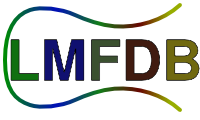show · test.text all knowls · up · search:

$\Aut G$, $\operatorname{Aut} G$.

## Math ∑ sign

A citation [MR:12345, arXiv:1234.5678, 1234345678].

This is a #test! $$1+ \frac{2}{4 5} + \frac{1}{x^2} + \dots \tag{SEQ}$$

Modstar: $\modstar{\Z}{q \Z}$

The 'test.nonexisting' Zeta function has [abc] $\leftrightarrow$ $$\leftrightarrow$$ $$\Leftrightarrow$$

• functional equation
• implies analytic continuation
• euler product

The analytic continuation for the $\zeta(s)$ function comes from

$$\Gamma(s)\zeta(s) = \int_0^{\infty}x^{s-1}e^{-x}dx$$

## More markdown examplesSomething something Google than Yahoo or MSN.

• emphasis or emphasis
• strong emphasis or strong emphasis Some text with some code inside

• An item in a bulleted (unordered) list

• A subitem, indented with 4 spaces
• Another item in a bulleted list

horizontal line

horizontal line

horizontal line

horizontal line

horizontal line

Test

test

Authors:
Knowl status:
• Review status: beta
• Last edited by Andrew Sutherland on 2022-09-09 17:02:44
Referred to by:
History: Differences